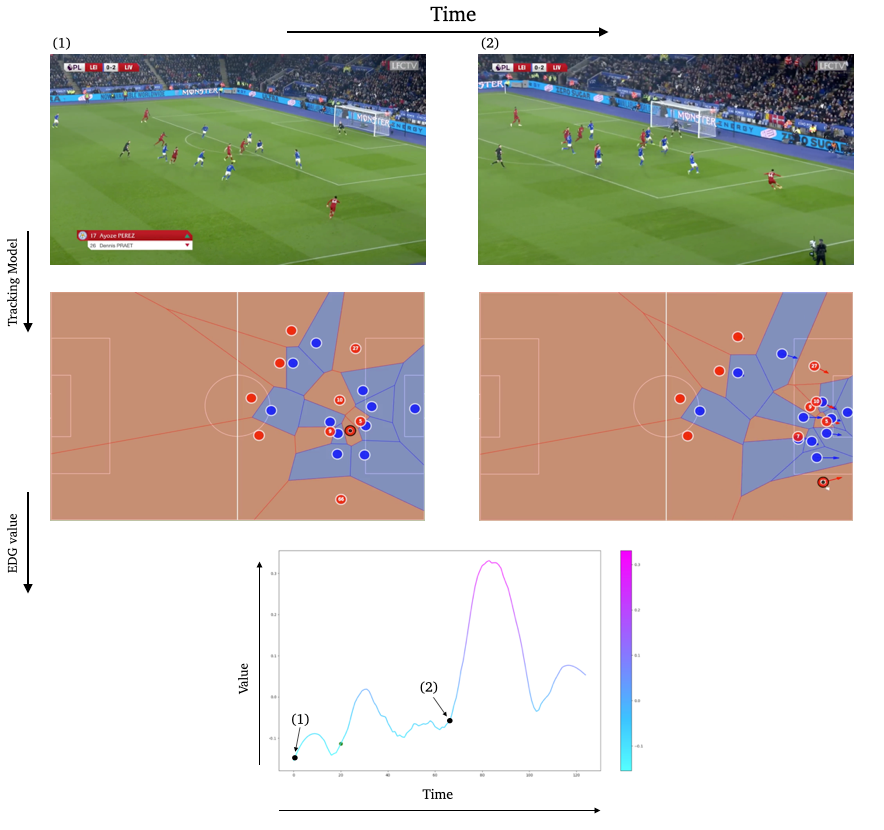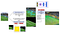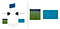# Narya — Tracking and Evaluating Soccer Players

Copy from a previous blog post, written in 12/2020.Evolution of our Tracking and EDG model over time. The EDG model learns to capture actions with potential in a very general manner, and compute such potential with the player coordinates our Tracking model gathers from the live Camera.

This blog post is the markdown version of a list of Jupyter Notebooks you can find inside Narya’s repository. This post allows to have each Notebook at the same place. It will probably be replaced by a Jupyter Book whenever I find the time and the solution to integrate them into this blog.

This project is also an evolution from a previous blog post.

We tried to make everything easy to reuse, we hope anyone will be able to:

• Use our datasets to train other models

# Narya

The Narya API allows you to track soccer player from camera inputs, and evaluate them with an Expected Discounted Goal (EDG) Agent. This repository contains the implementation of the following paper. We also make available all of our trained agents, and the datasets we used as well.

This Notebook’s goal is to allow anyone without any access to soccer data to produce its own and analyze them with powerful tools. We also hope that by releasing our training procedures and datasets, better models will emerge and make this tool better iteratively.

# Framework

Our library is split in 2: one part is to track soccer players, another one is to process these trackings and evaluate them. Let’s start by focusing on how to track soccer players.

# Installation

`git clone && cd narya && pip3 install -r requirements.txt`

Let’s start by importing some libraries and an image we will use during this Notebook:

# Tracking Soccer Players(a) The ET model computes the position of each entity. It then passes the coordinates to the online tracker to: (1): Compute the embedding of each player, (2): Warp each coordinate with the homography from HE. (b) The HE starts by doing a direct estimation of the homography. Available keypoints are then predicted and used to compute another estimation of the homography. Both predictions are used to remove potential outliers. © The REID model computes an embedding for each detected player. It also compares the IoU’s of each pair of players and applies a Kalman Filter to each trajectory.

# Players detections

The Player Detection model :

takes an image as input, and predicts a list of bounding boxes associated with a class prediction (Player or Ball) and a confidence value. The model is based on a Single Shot MultiBox Detector (SSD), with an implementation from GluonCV.

You can easily:

• Load the model

We tried to keep a similar architecture for each model, even with a different framework. For example, each model deals on itself with image preprocessing, reshaping, and so on: a simple `__call__` is enough.

Let’s start by importing a tracking model:

and load our pre-trained weights:

Note: When a `TrackerModel` gets instantiate more than once, weights won’t load successfully. Make sure to restart the kernel in this case.

You can now easily use this model to make predictions on any soccer related images. Let’s try it on our example:

Now that we have players’ coordinates (and the ball position), we need to be able to transform them into 2D coordinates. This means finding the homography between our input image and a 2D representation of the field:(left) Example of a planar surface (our soccer field) viewed by two camera positions: the 2D field camera and the Live camera. Finding the homography between them allows to produce 2D coordinates for each player. (right) Example of points correspondences between the 2D Soccer Field Camera and the Live Camera.

# Homography Estimations

We developed 2 methods to ensure more robust estimations of the current homography. The first one is a direct prediction, and the second one computes the homography from the detection of some particular keypoints. Let’s start with the direct prediction:

The model is based on a Resnet-18 architecture and takes images of shape (280,280). It was implemented with Keras. Let’s review its architecture, which is kept the same for each model, no matter its framework.

Each model is created with:

• The shape of its input

It then creates a model and a preprocessing function:

Each model then has the same call function:

Let’s apply this direct homography estimation to our example. This can be done easily, exactly like the tracking model:

Let’s load a “template” image, a 2D view of the field. This is the image to which we will apply our predicted homography:

and let’s make it easier to display on another image:

Now, let’s import some utils functions, and warp our template with our predicted homography:

You can also merge the warped template with your example:

Notes: Usually, this homography is only used to compute the coordinates of each player.

Our second approach is based on keypoints detection:

we predict p masks, each mask representing a particular keypoint on the field. The homography is computed knowing the coordinates of available keypoints on the image, by mapping them to the keypoints coordinates of the 2-dimensional field. The model is based on an EfficientNetb-3 backbone on top of a Feature Pyramid Networks (FPN) architecture to predict each keypoint’s mask. We implemented our model using Segmentation Models.

Again, let’s start by quickly creating our model and making some predictions:

Here, we display a concatenation of each keypoints we predicted. Now, since we know the “true” coordinates of each of them, we can precisely compute the related homography parameters.

Notes: We explain here how the homography parameters are computed. This is a Supplementary Material from our paper and, therefore, can be skipped.

We assume 2 sets of points (x1,y1) and (x2,y2) both in ℝ^2, and define X_i as:

We define the planar homography H that relates the transformation between the 2 planes generated by X_1and X_2 as :

where we assume h_33=1 to normalize H and since H only has 88 degrees of freedom as it estimates only up to a scale factor. The equation above yields the following 2 equations:

that we can rewrite as :

or more concisely:

where

We can stack such constraints for n pair of points, leading to a system of equations of the form A*h=0 where A is a 2n×8 matrix. Given the 8 degrees of freedom and the system above, we need at least 8 points (4 in each plan) to compute an estimation of our homography.

This is the method we use to compute the homography from the keypoints prediction.

Let’s do it and predict an homography from these keypoints:

and if we merge them:

`test = merge_template(image/255.,cv2.resize(pred_warp, (1024,1024)))visualize(image = test)`

# ReIdentification

Finally, we need to be able to say if one player from the first frame is the same in another frame. We use three tools to do so:

• a Kalman filter, to remove outliers

Our last model deals with the embedding part. Once again, even as a torch model, it can be loaded and used as the rest.

Let’s start by cropping the image of a player:

`x_1 = int(bbox)y_1 = int(bbox)x_2 = int(bbox)y_2 = int(bbox)print(x_1,x_2,y_1,y_2)resized_image = cv2.resize(image,(512,512))plt.imshow(resized_image[y_1:y_2,x_1:x_2])`

Now, we can create and call our model:

In the next section, we will see how to use all of these models together to track players on a video.

# Online Tracking

Given a list of images, we want to track players and the ball and gather their trajectories. Our model initializes several tracklets based on the detected boxes in the first image. In the following ones, the model links the boxes to the existing tracklets according to:

1. their distance measured by the embedding model,

When the entire list of images is processed, we compute a homography for each image. We then apply each homography to the player’s coordinates.

# Inputs

Let’s start by gathering a list of images:

First, we initialize a 2d Field template:

and then we create our list of images:

We can vizualize the first image of our list:

`image_0,image_25,image_50 = img_list,img_list,img_listprint("Image shape: {}".format(image_0.shape))visualize(image_0=image_0,            image_25=image_25,            image_50=image_50)`

# Football Tracker

We first need to create our tracker. This object gathers every one of our models:

We now only have to call it on our list of images. We manually remove some failed homography estimation, at frame ∈25,…,30 by adding `skip_homo = [25,26,27,28,29,30]` into our call.

`trajectories = tracker(img_list,split_size = 512, save_tracking_folder = 'test_outputs/',                        template = template,skip_homo = [25,26,27,28,29,30])`

Let’s check the same images as before but with the tracking information:

`imgs_ordered = []for i in range(0,51):    path = 'test_outputs/test_' + '{:05d}'.format(i) + '.jpg'    imgs_ordered.append(path)    img_list = []for path in imgs_ordered:    if path.endswith('.jpg'):        image = cv2.imread(path)        image = cv2.cvtColor(image, cv2.COLOR_BGR2RGB)        img_list.append(image)image_0,image_25,image_50 = img_list,img_list,img_listprint("Image shape: {}".format(image_0.shape))visualize(image_0=image_0,            image_25=image_25,            image_50=image_50)`

You can also easily create a movie of the tracking data, and display it:

`import imageioimport progressbarwith imageio.get_writer('test_outputs/movie.mp4', mode='I',fps=20) as writer:    for i in progressbar.progressbar(range(0,51)):        filename = 'test_outputs/test_{:05d}.jpg'.format(i)        image = imageio.imread(filename)        writer.append_data(image)`

# Process trajectories

We now have raw trajectories that we need to process. Fist, you can do several operations to ensure that the trajectories are functional:

• Delete an id at a specific frame

These operations are simple to do with some of our functions from `narya.utils.tracker`:

Here, let’s assume we don’t have to perform any operations, and directly process our trajectories into a Pandas Dataframe.

First, we can save our raw trajectory with

`import jsonwith open('trajectories.json', 'w') as fp:    json.dump(trajectories, fp)`

Let’s start by padding our trajectories with np.nan and building a dict. for our dataframe:

`import jsonwith open('trajectories.json') as json_file:    trajectories = json.load(json_file)from narya.utils.tracker import build_df_per_iddf_per_id = build_df_per_id(trajectories)`

We now fill the missing values, and apply a filter to smooth the trajectories:

`from narya.utils.tracker import fill_nan_trajectoriesdf_per_id = fill_nan_trajectories(df_per_id,5)from narya.utils.tracker import get_full_resultsdf = get_full_results(df_per_id)`

# EDG

Now that we have some tracking data, it is time to evaluate them.

# Theoretical framework

We assume s_t∈S is the state of the game at time t. It may be the positions of each player and the ball for example. Given an action a∈A (e.g. a pass, a shot,etc), and a state s′∈S, we note:

the probability

of getting to state s′ from s following action a.

Applying actions over K time steps yields a trajectory of states and actions:

We denote r_t the reward given going from s_t to s_(t+1) (e.g. +1 if the team scores a goal). More importantly, the cumulative discounted reward along τ is defined as:

where γ∈[0,1] is a discount factor, smoothing the impact of temporally distant rewards.

A policy, π_θ, chooses the action at any given state, whose parameters, θ, can be optimized for some training objectives (such as maximizing R). Here, a good policy would be a policy representing the team we want to analyze in the right manner. The Expected Discounted Goal (EDG), or more generally, the state value function, is defined as:

It represents the discounted expected number of goals the team will score (or concede) from a particular state. To build such a good policy, one can define an objective function based on the cumulative discounted reward:

and seek the optimal parametrization θ^∗ that maximize J(θ):

To that end, we can compute the gradient of such cost function (using a log probability trick), we can show that we have the following equality:

∇_θJ(θ) to update our parameters with θ←θ+λ∇θJ(θ). In our case, the evaluation of V_π and π_θ is done using Neural Networks, and θ represents the weights of such networks. At inference, our model will take the state of the game as input, and will output the estimation of the EDG.

# Implementation

Our EDG agent was implemented using the Google Football library. We trained our agent against bots and against itself until it became strong enough. Such an agent can be seen on this youtube video.

Let’s start by importing some libraries:

Notes: Google Football is not compatible with Tensorflow 2 yet. We have to downgrade it to use our agent.

Like the tracking models, our agent can easily be created:

# Loading and processing tracking data

First, we need to process our tracking data into a Google Football format. We built a few functions to do this.

Let’s load some tracking data from Liverpool:

`data = pd.read_csv('liverpool_2019.csv', index_col=('play', 'frame'))data['edgecolor'] = data['edgecolor'].fillna(0)data.tail()`

Let’s process them to add some features:

`from narya.utils.google_football_utils import _add_ball_coordinates, _add_possessiondata = data.rename(columns={'edgecolor':'id'})data_test = _add_ball_coordinates(data,id_ball=0)data_test = _add_possession(data_test)data_test = data_test.rename(columns={'id':'edgecolor'})`

We can chose one game, and display the first frame:

`play =  'Leicester 0 -  Liverpool'df = data_test[data_test['play'] == play]df = df.set_index('frame')df['bgcolor'] = df['bgcolor'].fillna('black')df.tail()from narya.utils.vizualization import draw_framefig, ax, dfFrame = draw_frame(df,t=0,add_vector = False,fps=20)`

We can also add a Voronoi Diagram and velocity vectors on our field:

`from narya.utils.vizualization import add_voronoi_to_figfig, ax, dfFrame = draw_frame(df,t=0,fps=20)fig, ax, dfFrame = add_voronoi_to_fig(fig, ax, dfFrame)`

# Google Format

We now have to change our data into a google format. To do so, we need to change:

• the Ball positions and velocity

And transfer the coordinates in a different representation.

We can now plot an EDG map at t=1. It represents the location with the most potential on the field.

`map_value = agent.get_edg_map(observations['obs'],observations['obs_count'],79,57,entity = 'ball')from narya.utils.vizualization import add_edg_to_figfig, ax, dfFrame = draw_frame(df, t=1)fig, ax, edg_map = add_edg_to_fig(fig, ax, map_value)`

You can also plot the EDG value over time:

`from narya.utils.vizualization import draw_linefor indx,obs in enumerate(observations['obs']):    value = agent.get_value([obs])    observations['value'].append(value)df_dict = {    'frame_count':observations['frame_count'],    'value':observations['value']}df_ = pd.DataFrame(df_dict)fig, ax = draw_line(df_,1,20, smooth=True)`

# Training and datasets

Finally, we also release our datasets and models.

# Links:

You can find below the datasets we used for training. You can also find the trained models in the repository.

# Overview

Homography Dataset: The homography dataset is made of pair of images,matrix in a .jpg,.npy format. The matrix is the homography associated with the image. They are normalized, meaning that homography[2,2] == 1.

Keypoints Dataset: We give here pair of images,xml file. The .xml files are made of the coordinates of each available keypoints on the image. We built utils function to read these files, and do so automaticaly in our Dataset class.

Tracking Dataset: Pair of images,xml files in a VOC format.

# Training

Finally, we give here a quick tour of our training scripts.

We start by creating a model:

We then create a loss function and an optimizer:

We can easily build a Dataset and a Dataloader (handling batches):

Finally, easily launch a training with:

Building http://flaneer.com, robots, and trying to solve one equation at a time

## More from Paul Garnier

Building http://flaneer.com, robots, and trying to solve one equation at a time